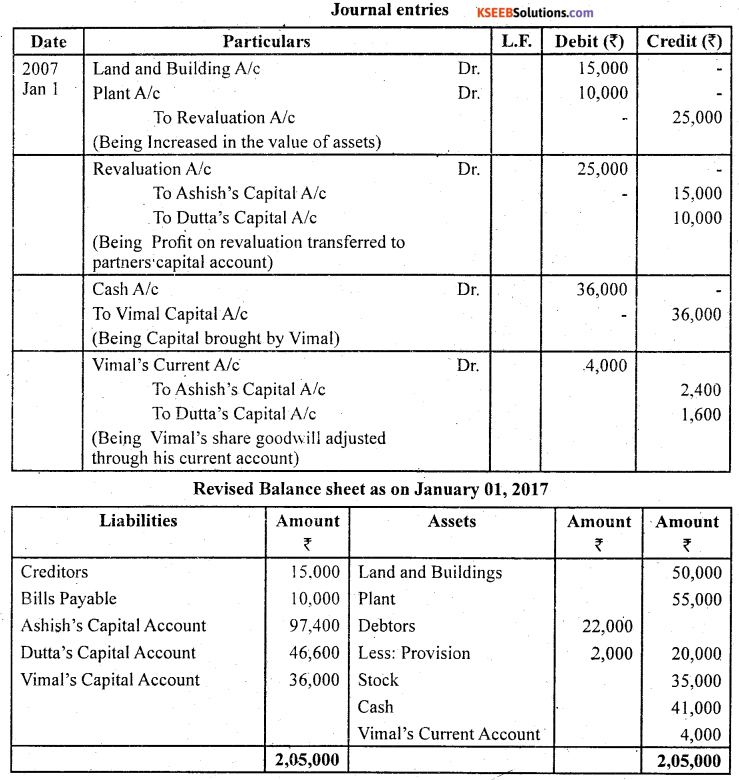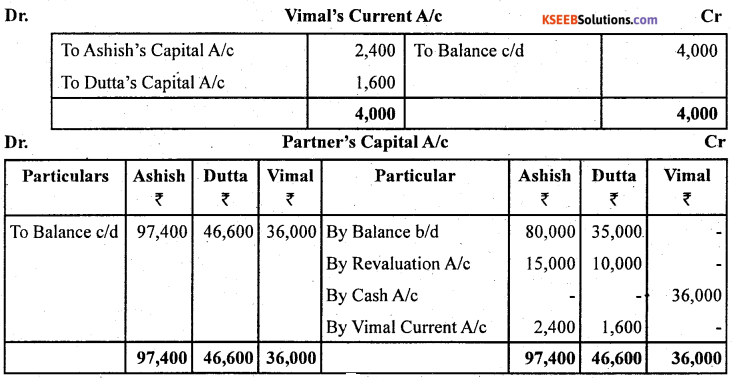# 2nd PUC Accountancy Question Bank Chapter 3 Reconstruction of a Partnership Firm – Admission of a Partner

## Karnataka 2nd PUC Accountancy Question Bank Chapter 3 Reconstruction of a Partnership Firm – Admission of a Partner

### 2nd PUC Accountancy Reconstruction of a Partnership Firm – Admission of a Partner NCERT Text Book Questions and Answers

2nd PUC Reconstruction of a Partnership Firm – Admission of a Partner Short Answer Questions With Answers

Question 1.
Identify various matters that need adjustments at the time of admission of a new partner.
The important points which require attention at the time of admission of a new partner:

• New profit sharing ratio;
• Sacrificing ratio;
• Valuation and adjustment of goodwill;
• Revaluation of assets and Reassessment of liabilities;
• Distribution of accumulated profits (reserves); and

Question 2.
Why it is necessary to ascertain new profit sharing ratio even for old partners when a new partner is admitted?
When new partner is admitted he acquires his share in the profits from the old partners. In other words, on the admission of a new partner, the old partners sacrifice a share of their profit in favour of the new partner. But, what will be the share of new partner and how he will acquire it from the existing partners is decided mutually among the old partners and the new partner.

On admission of a new partner, the profit sharing ratio among the old partners will change . keeping in view their, respective contribution to the profit sharing ratio of the incoming partner. Hence, there is a need to ascertain the new profit sharing ratio among all the partners.Question 3.
What is sacrificing ratio? Why is it calculated?

• Sacrifice ratio is the ratio sacrificed by the old partners to the new partner.
• Sacrifice ratio is calculated in order to distribute the goodwill brought in cash by the new partner on account of admission.

Question 4.
On what occasions sacrificing ratio is used?
The new partner is required to compensate the old partner’s for their loss of share in the super profits of the firm for which he brings in an additional amount known as premium or goodwill. This amount is shared by the existing partners in the ratio in which they forego their shares in favour of the new partner which is called sacrificing ratio.

Question 5.
If some goodwill already exists in the books and the new partner brings in his share of goodwill in cash, how will you deal with existing amount of goodwill?
When goodwill already exists in books: It is quite possible that when a new partner brings in his share of goodwill in cash, some amount of goodwill already exists in books. In that case, after crediting the old partners by the amount of goodwill brought in by the new partner, the existing goodwill must be written off by debiting the old partners in their old profit sharing ratio. But, if it is decided that the goodwill may continue to appear in the books at its old value, the amount to be brought in by new partner will have to be proportionately reduced

Question 6.
Why there is need for the revaluation of assets and liabilities on the admission of a partner?
Revaluation Method: This method is followed when the new partner does not bring in his share of goodwill in cash. In such a situation, the goodwill account is raised in the books of account by crediting the old partners in the old profit sharing ratio. When goodwill account is to be raised in the books of account there are two possibilities,
(a) No goodwill appears in books at the time of admission, and

(a) When no goodwill exists in the books: When no goodwill exists in the books at the time of the admission of a new partner, the goodwill account must be raised at its full value. This can be done by debiting goodwill account with its full value and crediting the old partners’ capital accounts in their profit sharing ratio. The journal entry will be:
Goodwill A/c Dr.
To Old Partners’Capitals A/c (individually)
(Goodwill raised at full value in the old ratio)
The goodwill thus raised shall appear in the balance”sheet of the firm at its full value.

2nd PUC Reconstruction of a Partnership Firm – Admission of a Partner Long Answer Questions With Answers

Question 1.
Do you advise that assets and liabilities must be revalued at the time, of admission of a partner? If so, why? Also describe how is this treated in the book of account?
Adjustments for Revaluation of. Assets and Reassessment of Liabilities: If, at the time of admission of a partner, the assets and liabilities are revalued or some asset or liability is found unrecorded, necessary adjustments are made through the Revaluation Account. Any gain or loss arising from such exercise shall be distributed among the old partner’s in their old profit sharing ratio.

The accounting treatment in the book of accounts are – in case of increase in the value of assets It should be recorded on credit side of revaluation account arid in case of decrease of assets recorded on debit side of revaluation account. In case of increase of liabilities recorded on debit side of revaluation account. In case of decrease in the value of liabilities and It should be recorded on credit side of revaluation accountQuestion 2.
What is goodwill? What factors affect goodwill?
A well-established business develops an advantage of good name, reputation and wide business connections. This helps the business to earn more profits as compared to a newly set up business. In accounting, the monetary value of such advantage is known as “goodwill”.

The main factors affecting the value of goodwill are as follows:

• Nature of business: A firm that produces high value added products or having a stable demand is able to earn more profits and therefore has more goodwill.
• Location: If the business is centrally located or is at a place having heavy customer traffic, the goodwill tends to be high.
• Efficiency of management: A well-managed concern usually enjoys the advantage of . ‘ high productivity and cost efficiency. This leads to higher profits and so the value of goodwill will also be high.
• Market situation: The monopoly condition or limited competition enables the concern to earn high profits which leads to higher value of goodwill.
• Special advantages: The firm that enjoys special advantages like import licences, low rate and assured supply of electricity, long-term contracts for supply of materials, well- known collaborators, patents, trademarks, etc. enjoy higher value of goodwill.

Question 3.
Explain various methods of valuation of goodwill.
Goodwill is an intangible asset it is very difficult to accurately calculate its value. Various methods have been advocated for the valuation of goodwill of a partnership firm, Goodwill calculated by one method may differ from the goodwill.
Calculated by another method. Hence, the method by which goodwill is to be calculated, may be specifically decided between the existing partners and the incoming partner.
The important methods of valuation of goodwill are as follows:

1. Average Profits Method.
2. Supper Profits Method
3. Capitalisation Method

1. Average Profits Method: Under this method, the goodwill is valued at agreed number of . ‘years’ purchase of the average profits of the past few years. It is based on the assumption that a new business will not be able to earn any profits during the first few years of its operations.

2. Supper Profits Method : The basic assumption in the average profits (simple or weighted) method of calculating goodwill is that if a new business is set up, it will not be able to earn any profits during the first few years of its operations. Hence, the person who purchases an existing business has to pay in the form of goodwill a sum equal to the total profits he is likely to receive for the first‘few years’.

3. Capitalisation Methods: Under this method the goodwill can be calculated in two ways:
(a) by capitalizing the average profits, or
(b) by capitalizing the super profits.

(a) Capitalisation of Average Profits: Under this method, the value of goodwill is ascertained by deducting the actual capital employed (net assets) in the business from the capitalized value of the average profits on the basis of normal rate of return. This involves the following steps:

1. Ascertain the average profits based on the past few years’ performance.
2. Capitalize the average profits on the basis of the normal rate of return to ascertain the capitalised value of average profits as follows:
Average Profits × 100/Normal Rate of Return
3. Ascertain the actual capital employed (net assets) by deducting outside liabilities from the total assets (excluding goodwill).
Capital Employed = Total Assets (excluding goodwill) – Outside Liabilities
4. Compute the value of goodwill by deducting net assets from the capitalised value of average profits, i.e. (2) – (3).

Question 4.
If it is agreed that the capital of all the partners should be proportionate to the new profit sharing ratio, how will you work out the new capital of each partner?
The total capital of the firm may clearly be specified and it is agreed that the capital of each partner should be proportionate to his share in profits. In such a situation each partner’s capital (including the new partner’s capital to be brought by him) is calculated on the basis of his share in profits.

By bringing in additional amount or withdrawal of excess amount, the final capital of each partner can be brought up to the required level.

It may be noted that subject to agreement among the partners, surplus or deficiency in each old partners’ capital accounts can also be taken care of simply by transfer to their respective current accounts.Question 5.
Explain how will you deal with goodwill when new partner is not in a position to bring his share of goodwill in cash.
The incoming partner who acquires his share in the profits of the firm from the existing partners brings in some additional amount to compensate them for loss of their share in super profits. It is termed as his share of goodwill (also called premium). Alternatively he may agree that goodwill account be raised in the books of the firm by giving the necessary credit to the old partners. Thus, when a new partner is admitted, goodwill can be treated in two ways:

2. By Revaluation Method.

Premium Method: This method is followed when the new partner pays his share of goodwill in cash. The amount of premium brought in by the new partner is shared by the existing partners in their ratio of sacrifice.

Question 6.
Explain various methods for the treatment of goodwill on the admission of a new partner?
Over a period of time, a well-established ‘business develops an advantage of good name, reputation and wide business connections. This helps the business to earn more profits as compared to a newly set up business. In accounting, the monetary value of such advantage is known as “goodwill”.

Methods of valuation of Goodwill: Since goodwill is an intangible asset it is very difficult to accurately calculate its value. Various methods have been advocated for the valuation of goodwill of a partnership firm. Goodwill calculated by one method may differ from the goodwill calculated by another method. Hence, the method by which goodwill is to be calculated, may be specifically decided between the existing partners and the incoming partner.

The important methods of valuation of goodwill are as follows:

1. Average Profits Method
2. Supper Profits Method’
3. Capitalisation Method

1. Average Profits Method: Under this method, the goodwill is valued at agreed number of ‘years’ purchase of the average profits of the past few years. It is based on the assumption that a new business will not be able to earn any profits during the first few years of its operations.

2. Supper Profits Method: The basic assumption in the average profits (simple or weighted) method of calculating goodwill is that if a new business is set up, it will not be able to earn any profits during the first few years of its operations. Hence, the person who purchases an existing business has to pay in the form of goodwill a sum equal to the total profits he is likely to receive for the first‘few years’.

3. Capitalisation Methods: Under this method the goodwill can be calculated in two ways:

1. by capitalizing the average profits, or
2. by capitalizing the super profits.

(a) Capitalisation of Average Profits: Under this method, the value of goodwill is ascertained by deducting the actual capital employed (net assets) in the business from the capitalized value of the average profits on the basis of normal rate of return. This ” involves the following steps:

1. Ascertain the average profits based on the past few years’ performance.
2. Capitalize the average profits on the basis of the normal rate of return to ascertain the capitalised value of average profits as follows:
Average Profits × 100/Normal Rate of Return
3. Ascertain the actual capital employed (net assets) by deducting outside liabilities ‘ from the total assets (excluding goodwill).
Capital Employed = Total Assets (excluding goodwill) – Outside Liabilities
4. Compute the value of goodwill by deducting net assets from the capitalised value of average profits, i.e. (2) – (3).

Question 7.
How will you deal with the accumulated profits and losses and reserves on the admission of a new partner?
A firm may have accumulated profits not yet transferred to capital accounts of the partners. These are usually in the firm of general reserve, reserve fund and/or Profit and Loss Account balance. The new partner is not entitled to have any share in such accumulated profits. These are distributed among the partners by transferring it to their capital accounts in old profit sharing ratio.

Similarly, if there are some accumulated losses in the form of a debit balance of profit and loss account appearing in the balance sheet of the firm. A remote possibility, the same should also be transferred to the old partners’ capital accounts

Question 8.
At what figures the value of assets and liabilities appear in the books of the firm after revaluation has been due. Show with the help of an imaginary balance sheet.
At the time of admission of a new partner, it is always desirable to ascertain whether the assets of the firm are shown in books at their current values. In case the assets are overstated or understated, these are revalued. Similarly, a reassessment of the liabilities is also done so that these are brought in the books at their correct values.

At times there may also be some unrecorded assets and liabilities of the firm. These also have to be brought into the books of the firm. For this purpose the firm has to prepare the Revaluation Account. The gain or loss on revaluation of each asset and liability is transferred to this account and finally its balance is transferred to the capital accounts of the old partners in their old profit sharing ratio.

In other words, the revaluation account is credited with increase in the value of each asset and decrease in its liabilities because it is a gain and is debited with decrease in the value of assets and increase in its liabilities is debited to revaluation account because it is a loss. Similarly unrecorded assets are credited and unrecorded liabilities are debited to the revaluation account.

If the revaluation account finally shows a credit balance then it indicates net gain and if there is a debit balance then it indicates net loss. Which will be transferred to the capital accounts of the old partners in old ratio.2nd PUC Reconstruction of a Partnership Firm – Admission of a Partner Numerical Questions and Answers

Question 1.
A and B were partners in a firm sharing profits and losses in the ratio of 3:2. They admit C into the partnership with 1/6 share in the profits. Calculate the new profit sharing ratio?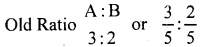C admits for $$\frac { 1 }{ 6 }$$ share of new profit in new firm.
Let new firm’s profit =1
Remaining share of A and B in the new firm = 1 – C’s share.
$$=1-\frac{1}{6}=\frac{5}{6}$$
New Ratio = Old Ratio × Remaining Share of A and B
$$A=\frac{3}{5} \times \frac{5}{6}=\frac{15}{30}$$ $$, B=\frac{2}{5} \times \frac{5}{6}=\frac{10}{30}$$
A : B : C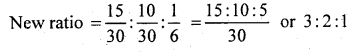Question 2.
A, B, C were partners in a firm sharing profits in 3:2:1 ratio. They admitted D for 10% profits. Calculate the new profit sharing ratio?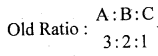D admits for $$\frac { 10 }{ 100 }$$ share in the new firm. Let new firm’s profit = 1
Remaining share of A, B and C in new firm = 1 – D’s share
$$=1-\frac{10}{100}=\frac{90}{100}=\frac{9}{10}$$
New Ratio Old Ratio × Remaining Share of A, H and C in new firm
A = $$\frac{3}{6} \times \frac{9}{10}=\frac{27}{60}$$, B = $$\frac{2}{6} \times \frac{9}{10}=\frac{18}{60}$$, C = $$\frac{1}{6} \times \frac{9}{10}=\frac{9}{60}$$
A:B:C:D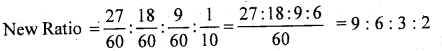Question 3.
X and Y are partners sharing profits in 5 : 3 ratio admitted Z for share which he acquired equally for X and Y. Calculate new profit sharing ratio?
X : Y Old Ratio = 5:3
Z admits for $$\frac { 1 }{ 10 }$$ share in the new firm.
X and Y each sacrifice = $$\frac{1}{10} \times \frac{1}{2}=\frac{1}{20}$$
New Ratio = Old Ratio – Sacrificing Ratio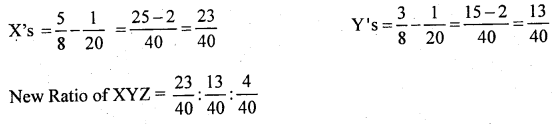Question 4.
A, B and C are partners sharing profits in 2 : 2 : 1 ratio admitted D for 1/8 share which he acquired entirely from A. Calculate new profit sharing ratio?
A : B : C’s Old Ratio = 2 : 2 : 1 = $$\frac{2}{5}: \frac{2}{5}: \frac{1}{5}$$
D admits for $$\frac { 1 }{ 8 }$$ share in new firm, which he takes from A.
Here only A will sacrifice.
New Ratio = Old Ratio – Sacrificing Ratio ⇒ A = $$\frac{2}{5}-\frac{1}{8}=\frac{16-5}{40}=\frac{11}{40}$$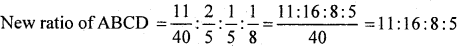Question 5.
P and Q are partners sharing profits in 2:1 ratio. They admitted R into partnership giving him 1/5 share which he acquired from P and Q in 1:2 ratio. Calculate new profit sharing ratio?
P:Q’s Old ratio = 2:1 = $$=\frac{2}{3}: \frac{1}{3}$$
R admits for $$\frac { 1 }{ 5 }$$ share in the new firm which he takes from $$\frac { 1 }{ 3 }$$ from P and $$\frac { 2 }{ 3 }$$ from Q.
P’s sacrifice = R’s share $$\times \frac{1}{3}=\frac{1}{5} \times \frac{2}{3}=\frac{2}{15}$$
New Ratio = Old Ratio – Sacrificing Ratio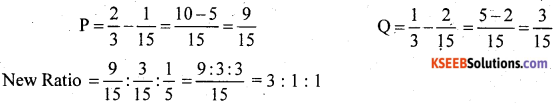Question 6.
A, B and C are partners sharing profits in 3 : 2 : 2 ratio. The admitted D as a new partner for 1/5 share which lie acquired from A, B and C in 2 : 2 : 1 ratio respectively. Calculate new profit sharing ratio?
A: B : C’s Old Ratio : 3 : 2 : 2
D admits for $$\frac { 1 }{ 5 }$$ share in the new firm which he takes $$\frac { 1 }{ 5 }$$ in the ratio 2:2:1 from A, B and C.
A’s sacrifice = D’s share × $$\frac { 2 }{ 5 }$$ B’s sacrifice = D’s share × $$\frac { 2 }{ 5 }$$ C’s share = D’s share × $$\frac { 2 }{ 5 }$$
New Ratio = Old Ratio – Sacrificing Ratio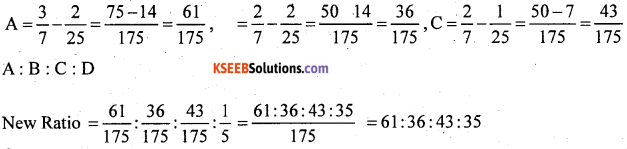Question 7.
A and B were partners in a firm sharing profits in 3 : 2 ratio. They admitted C for 3/7 share which he took 2/7 from A and 1/7 from B. Calculate new profit sharing ratio?
A:B Old Ratio = 3: 2 or $$\frac{3}{5}: \frac{2}{5}$$
C Admitted for $$\frac{3}{7}^{\text {th }}$$ share in the new firm
A’s sacrifice = $$\frac { 2 }{ 7 }$$ ⇒ B’s sacrifice = $$\frac { 1 }{ 7 }$$
New Ratio = Old Ratio – Sacrificing Ratio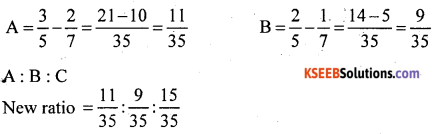Question 8.
A, B and C were partners in a firm sharing profits in 3 : 3 : 2 ratio. They admitted D as a new partner for 4/7 profit. D acquired his share 2/ 7 from A. 1/ 7 from B and 1/ 7 from C. Calculate new profit sharing ratio?
Old Ratio =A:B:C 3:3:2
D admitted for $$\frac { 4 }{ 7 }$$ share of profit in newfirm.
D’s share = A’s sacrifice + B’s sacrifice + C’s sacrifice
$$\frac{4}{7}=\frac{2}{7}+\frac{1}{7}+\frac{1}{7}$$
New Ratio = Old Ratio – Sacrificing Ratio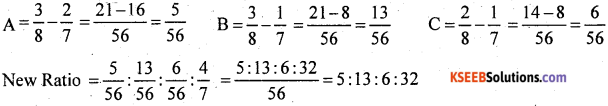Question 9.
Radha and Rukmini are partners in a firm sharing profits in 3:2 ratio. They admitted Gopi as a new partner. Radha surrendered 1/3 of her share in favour of Gopi and Rukmani surrendered 1/4 of her share in favour of Gopi. Calculate new profit sharing ratio?
Radha: Rukmani Old Ratio : 3 : 2
Radha surrendered in favour of Gopi = $$\frac { 1 }{ 3 }$$ of his share.
Rukmani surrendered in favour of Gopi = $$\frac { 1 }{ 4 }$$ of his share
Radha $$=\frac{3}{5} \times \frac{1}{3}=\frac{1}{5}$$ Rukumani $$=\frac{3}{5} \times \frac{1}{4}=\frac{1}{10}$$ ∴2:1
Sacrificing Ratio = Old Ratio x Surrender Ratio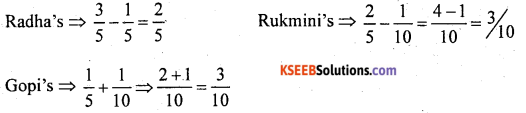New Ratio ⇒ 4:3:3

Question 10.
Singh, Gupta and Khan are partners in a firm sharing profits in 3:2:3 ratio. They admitted Jain as a new partner. Singh surrendered 1/3 of his share in favour of Jain: Gupta surrendered 1/4 of his share in favour of Jain and Khan surrendered 1/5 in favour of Jain. Calculate new profit sharing ratio?
Old Ratio = Singh : Gupta : Khan
= 3:2:3
$$=\frac{3}{8}: \frac{2}{8}: \frac{3}{8}$$
Singh surrender = $$\frac { 1 }{ 3 }$$ of his share
Gupta surrender = $$\frac { 1 }{ 4 }$$ of his share
Khan surrender = $$\frac { 1 }{ 5 }$$ of his share
Sacrificing ratio = old ratio × Surrender ratio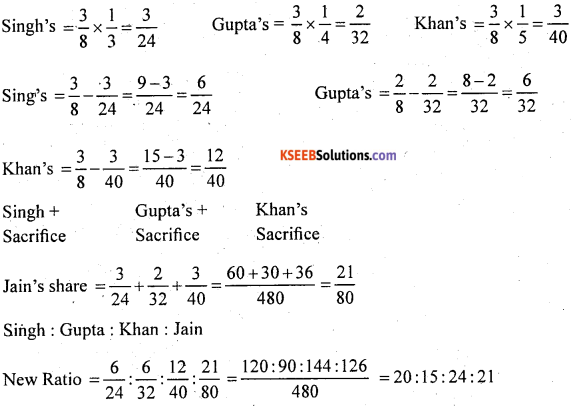Question 11.
Sandeep and Navdeep are partners in a firm sharing profits in 5:3 ratio. They admit C into the firm and the new profit sharing ratio was agreed at 4:2:1. Calculate the sacrificing ratio?
Sandeep : Navdeep Old Radio = 5 : 3 $$=\frac{5}{8}: \frac{3}{8}$$
Sandeep : Navdeep : C New Ratio = 4 : 2 : 1 = $$=\frac{4}{7}: \frac{2}{7}: \frac{1}{7}$$
Sacrificing Ratio = Old Ratio – New Ratio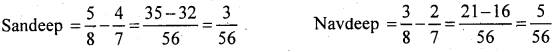Sandeep : Navdeep
Sacrificing $$=\frac{3}{56}: \frac{5}{56} 3: 5$$Question 12.
Rao and Swami are partners in a firm sharing profits and losses in 3 : 2 ratio. They admit Ravi as a new partner for 1/8 share in the profits. The new profit sharing ratio between Rao and Swami is 4:3. Calculate new profit sharing ratio and sacrificing ratio?
Rao : Swami Old Ratio -3:2
Ravi admit for $$\frac { 1 }{ 8 }$$ share of profit in the new firm.
Let the New Firm Profit =1
Combined share of Rao and Swami in the new firm
= 1 – Ravi’s share of profit. = $$1-\frac{1}{8}=\frac{7}{8}$$
New Ratio = Combined Share of Rao and Swami x Proportion of Rao and Swami in the combined share.
Rao $$=\frac{7}{8} \times \frac{4}{7}=\frac{28}{56}$$ Swami $$=\frac{7}{8} \times \frac{3}{7}=\frac{21}{56}$$
Rao : Swami : C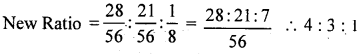Sacrificing Ratio = Old Ratio – New Ratio
Rao = $$\frac{3}{5}-\frac{4}{8}=\frac{24-20}{40}=\frac{4}{40}$$
Swami
Sacrificing Ratio = Rao : Swami = $$\frac{4}{40}: \frac{1}{40}$$ =4:1

Question 13.
Compute the value of goodwill on the basis of four years’ purchase of the average profits based on the last five years? The profits for the last five years were as follows:
2002 – 40,000
2003- 50,000
2004 – 60,000
2005 – 50,000
2006 – 60,000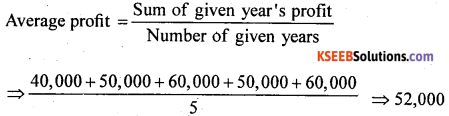Goodwill = Average Profit × Number of Year’s Purchases = 52,000 × 4 = ₹ 2,08,000

Question 14.
Capital employed in a business is 2,00,000. The normal rate of return on capital employed is 15%. During the year 2002, the firm earned a profit of ₹ 48,000. Calculate goodwill on the basis of 3 years purchase of super profit?
Capital Employed = ₹ 2,00,000
Actual Profit = 48,000
Normal Rate of Return 15%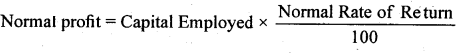$$=2,00,000 \times \frac{5}{100}=\mathrm{Rs} .30,000$$
Super profit = Actual profit – Normal profit = 48,000 – 30,000 = ₹ 18,000
Goodwill = Super Profi t × Number of Years Purchase = 18,000 × 3 = ₹ 54,000

Question 15.
The books of Rain and Bharat showed that the capital employed on 31.12.2002 was? 5,00,000 and the profits for the last 5 years :
2002 ₹ 40,000;
2003 ₹ 50,000;
2004 ₹ 55,000;
2005 ₹ 70,000 and
2006 ₹ 85,000.
Calculate the value of goodwill on the basis of 3 years purchase of the average super profits of the last 5 years assuming that the normal rate of return is 10%?
Sum of given year profit number of given years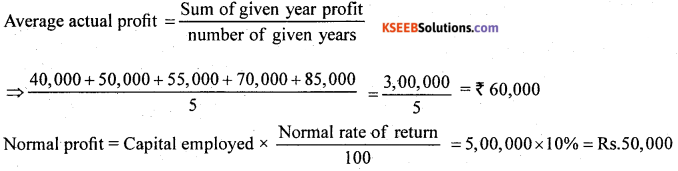Average Super Profit = Average Actual Profit – Normal Profit = 60,000 – 50,000 = ₹ 10,000
Goodwill = Average Super Profit × Number of year purchase
= 10,000 × 3 = ₹ 30,000

Question 16.
Rajan and Rajani are partners in a firm. Their capitals were Rajau ₹ 3,00,000; Rajani ₹ 2,00,000. During the year 2002, the firm earned a profit of ₹ 1,50,000. Calculate the value of goodwill of the firm assuming that the normal rate of return is 20%?

 Rajani’s Capital 3,00,000 Rajni’s Capital 2,00,000 Total Capital employed 5,00,000

Normal rate of return = 20%Goodwill = capitalised value – capital Employed = 7,50,000-5,00,000 = ₹ 2,50,000Question 17.
A business has earned average profits of ₹ 1,00,000 during the last few years. Find out the value of goodwill by capitalisation method, given that the assets of the business are ₹ 10,00,000 and its external liabilities are ₹ 1,80,000. The normal rate of return is 10%?
Capital Employed = Assets – External Liabilities
= 10,00,000 – 1,80,000 = ₹ 8,20,000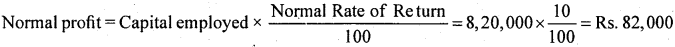Super Profit = Actual Profit – Normal Profit – 1,00,000 – 82,000 = ₹ 18,000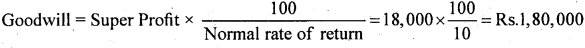Alternative Method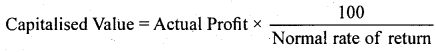Capitalised value = 1,00,000 × $$\frac { 10 }{ 100 }$$ = ₹ 1,00,000
Goodwill = Capitalised Value – Capital Employed
= 10,00,000 – 8,20,000 = ₹ 1,80,000

Question 18.
Verma and Sharma are partners in a firm sharing profits and losses in the ratio of 5:3. They admitted Ghosh as a new partner for 1/5 share of profits. Ghosh is to bring in ₹ 20,000 as capital and ₹ 4,000 as his share of goodwill premium. Give the necessary journal entries:
(a) When the amount of goodwill is retained in the business.
(b) When the amount goodwill is fully withdrawn.
(c) When 50% of the amount of goodwill is withdrawn.
(d) When goodwill is paid privately.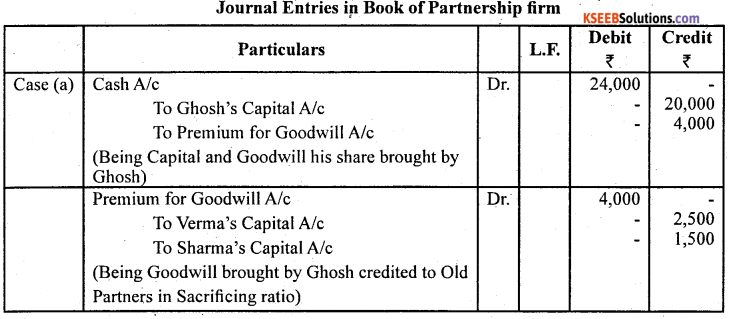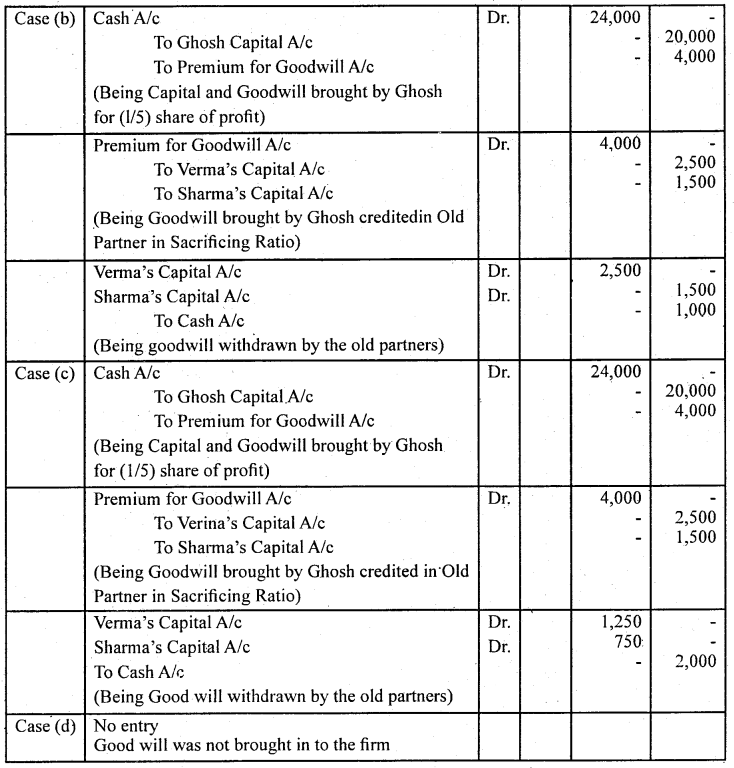Question 19.
A and B are partners in a firm sharing profits and losses in the ratio of 3 : 2. They decide to admit C into partnership with 1/4 share in profits. C will bring in ₹ 30,000 for capital and the requisite amount of goodwill premium in cash. The goodwill of the firm is valued at Rs, 20,000. The new profit sharing ratio is 2:1:1. A and B withdraw their share of goodwill. Give necessary journal entries?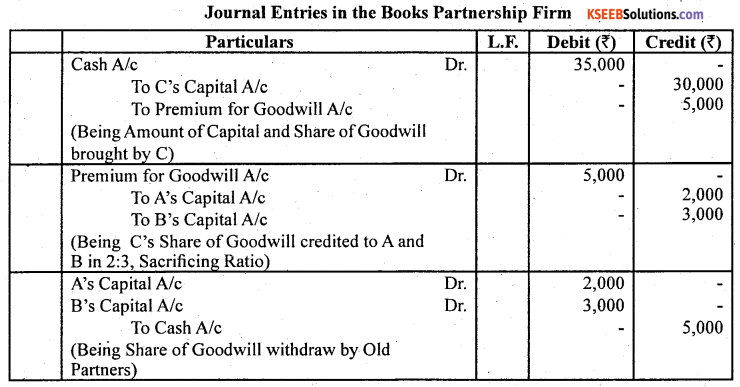Sacrificing Ratio = Old Ratio – New Ratio
A $$=\frac{3}{5}-\frac{2}{4}=\frac{12-10}{20}=\frac{2}{20}$$ B $$=\frac{2}{5}-\frac{1}{4}=\frac{8-5}{20}=\frac{3}{20}$$
A : B Sacrificing Ratio = $$\frac{2}{20}: \frac{3}{20}$$ =2:3 Goodwill of the firm = ₹ 20,000
C’s share of Goodwill = 20,000 x $$\frac { 1 }{ 4 }$$ = Rs.5,000
A will receive = 5,000 × $$\frac { 2 }{ 5 }$$ = 2,000 or 20,000 x $$\frac { 2 }{ 20 }$$ = 2,000
B will receive 20,000 × $$\frac { 3 }{ 20 }$$= 3,000Question 20.
Arti and Bharti are partners in a firm sharing profits in 3:2 ratio, They admitted Sarthi for 1/4 share in the profits of the firm. Sarthi brings ₹ 50,000 for his capital and ₹ 10,000 for his 1/4 share of goodwill. Goodwill already appears in the books of Arti and Bharti at ₹ 5,000. The new profit sharing ratio between Arti, Bharti and Sarthi will be 2:1:1. Record the necessary journal entries in the books of the new firm?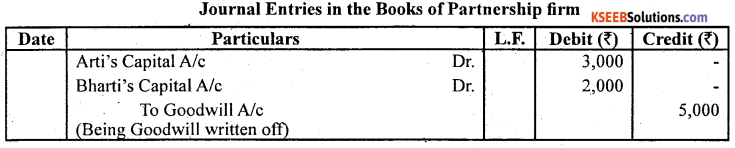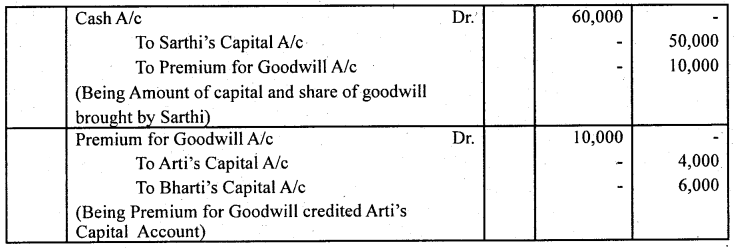Arti: Bharti Old Ratio 3:2
Sarthi admitted for $$\frac { 3 }{ 20 }$$ share in new firm Arti: Bharti: Sarthi
New ratio 2:1:1
Sacrificing Ratio = Old Ratio – New Ratio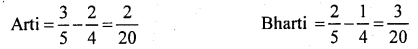Arti will receive = 10,000 × $$\frac { 2 }{ 5 }$$ Bharti will receive = 10,000 × $$\frac { 3 }{ 5 }$$ = 6000

Question 21.
X and Y are partners in a firm sharing profits and losses in 4 : 3 ratio. They admitted’ Z for 1/8 share. Z brought ₹ 20,000 for his capital and ₹ 7,000 for his 1/8 share of goodwill. Subsequently X, Y and Z decided to show goodwill in their books at ₹ 40,000. Show necessary entries in the books of X, Y and Z?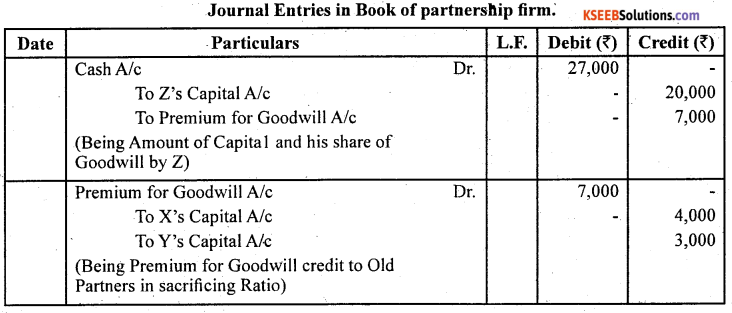Question 22.
Aditya and Balan are partners sharing profits and losses in 3 : 2 ratio. They Christopher for 1/4 share in The profits. The new profit sharing ratio agreed 2:1:1. Christopher brought ₹ 50,000 for his capital. His share of goodwill was agreed to at ₹ 15,000. Christopher could bring only ₹ 10,000 out of his share of goodwill. Record necessary journal entries in the books of the firm?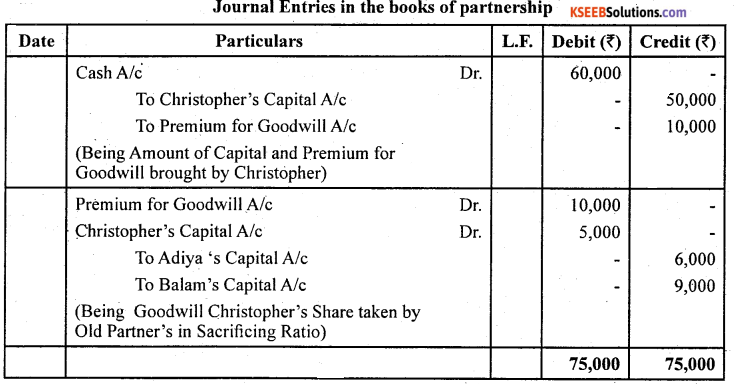Sacrificing Ratio = Old Ratio New Ratio
Aditya = $$\frac{3}{5}-\frac{2}{4}=\frac{12-10}{20}=\frac{2}{20}$$ Balam = $$\frac{2}{5}-\frac{1}{4}=\frac{8-5}{20}=\frac{3}{20}$$
Sacrificing ratio $$=\frac{2}{10}: \frac{3}{20}=\frac{2: 3}{20}$$ = 2:3

Question 23.
Amar and Samar were partners in a firm sharing profits and losses in 3:1 ratio. They admitted Kanwar for 1/4 share of profits. Kanwar could not bring his share of goodwill premium in cash. The Goodwill of the firm was valued at ₹ 80,000 on Kanwar’s admission. Record necessary journal entry for goodwill on Kanwar’s admission.
Amar: Samar.
Old Ratio 3:1
Kanwar admitted for $$\frac { 1 }{ 4 }$$ share of profit.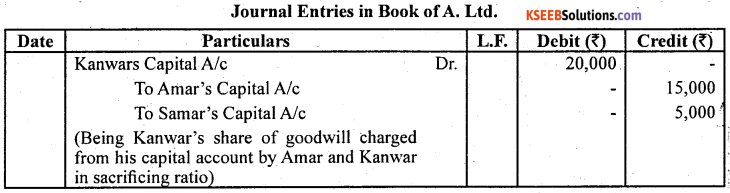New Firm’s Goodwill = ₹ 80,000
Kanwar’s Share of Goodwill = 80,000 × $$\frac { 1 }{ 4 }$$ 20,000Question 24.
Mohan Lai and Sohan Lai were partners in a firm sharing profits and losses in 3:2 ratio. They admitted Ram Lai for 1/4 share on 1.1.2003. It was agreed that goodwill of; the firm will be valued at 3 years purchase of the average profits of last 4 years which were
₹ 50,000 for 2003,
₹ 60,000 for 2004,
₹ 90,000 for 2005 and
₹ 70,000 for 2006.
Ram Lai did not bring his share of goodwill premium in cash. Record the necessary; journal entries in the books of the firm on Ram Lai’s admission when:
(a) Goodwill already appears in the books at ₹ 2,02,500.
(b) Goodwill appears in the books at ₹ 2,500.
(c) Goodwill appears in the books at ₹ 2,05,000.
Sum of profit ⇒ 50,000 + 60,000 + 90,000 + 70,000
⇒ 2,70,000
Average profit = $$\frac{2,70,000}{4}=\mathrm{Rs} .67,500$$
Goodwill Average Profit × No. of Years Purchases = 67,500 × 3 = 2,02,500
Ram Lal entered into the firm for 1/4 share of Profit.
Ram Lal’s share of goodwill = 2,02,500 × (1/4) = ₹ 50,625
Here sacrificing ratio of Mohan Lai and Sohan Lai will be equal to old ratio because new and sacrificing ratio is not given.
Mohan Lal will get Ram Lai’s Share of Goodwill × (3/5) = 50,625 × (3/5) = 10,125 × 3 = ₹ 30,375
Sohan Lai will Ramlal Share of Goodwill × (1/5) = 50,625 × (1/5)
= ₹ 10,125 × 2 = ₹ 20,250
Case (a)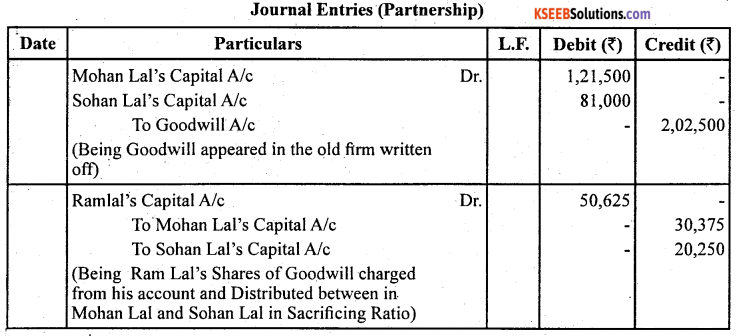Case (b)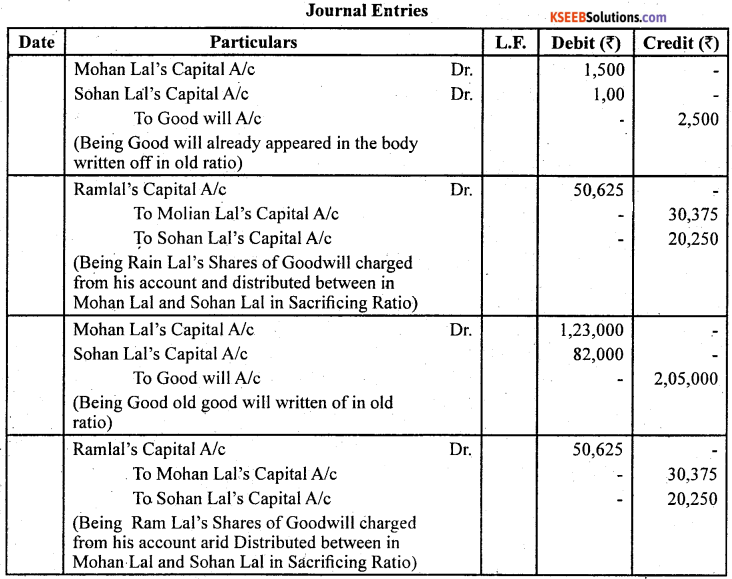Question 25.
Rajesh and Mukesh are equal partners in a firm. They admit Han into partnership and the new profit sharing ratio between Rajesh, Mukesh and Han is 4:3:2. On Han’s admission goodwill of the firm is valued at ₹ 36,000. Han is unable to bring his share goodwill premium in cash. Rajesh, Mukesh and Han decided not to show goodwill in their balance sheet. Record necessary journal entries for the treatment of goodwill on Han’s adtnission.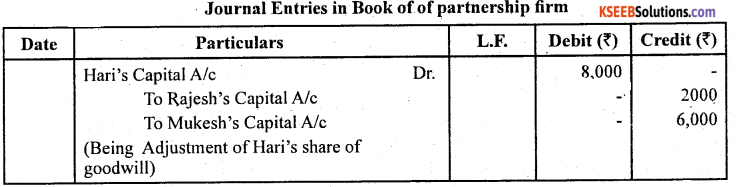Working notes :
1. Goodwill of a firm = 36,000 Hari’s share in goodwill
= Goodwill of firm × admitting Partner Share 36,000 × $$\frac { 2 }{ 9 }$$

2. Sacrificing Ratio = Old Ratio – New Ratio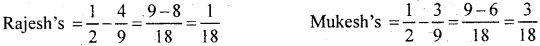Sacrificing Ratio between Rajesh and Mukesh 1:3Question 26.
Amar and Akbar are equal partners in a firm. They admitted Anthony as a new partner and the new profit sharing ratio is 4:3:2. Anthony could not bring this share of goodwill ₹ 45,000 in cash. It is decided to do adjustment for goodwill without opening goodwill account. Pass the necessary journal entry for the treatment of goodwill?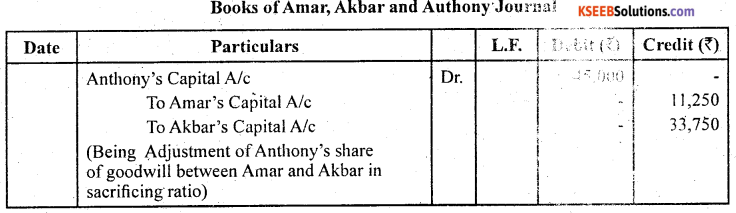Working notes:
1. Sacrificing Ratio = Old Ratio – New Ratio
Amar’s sacrificing ratio = $$\frac{1}{2}-\frac{4}{9}=\frac{9-8}{18}=\frac{1}{18}$$
Akbar’s sacrificing ratio = $$\frac{1}{2}-\frac{3}{9}=\frac{9-6}{18}=\frac{3}{18}$$
Sacrificing Ratio between Amar and Akbar =1:3.

Question 27.
Given below is the Balance Sheet of A and B, who are carrying on partnership business on 31.12.2016. A and B share profits and lossess the ratio of 2:1.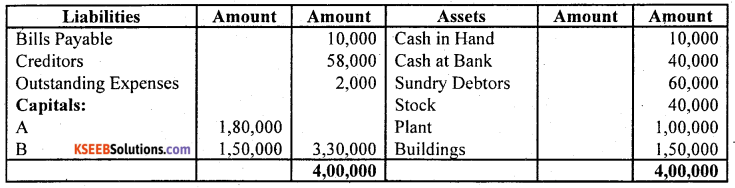C is admitted as a partner on the date of the balance sheet on the following terms:

1. C will bring in ₹ 1,00,900 as his capital and ₹ 60,000 as his share of goodwill for 1/4 share in the protits.
2. Plant is to be appreciated to ₹ 1,20,000 and the value of buildings is to be appreciated by 10%.
3. Stock is found over valued by ₹ 4,000.
4. A provision for bad and doubtful debts is to be created at 5% of debtors.
5. Creditors were unrecorded to the extent of ₹ 1,000.

Pass the necessary journal entries, prepare the revaluation account and partners’ capital accounts, and show the Balance Sheet after the admission of C.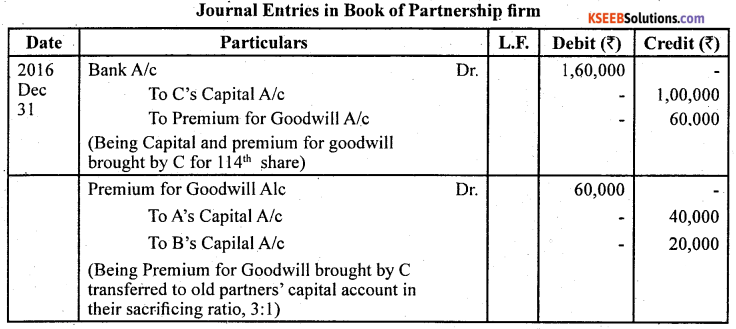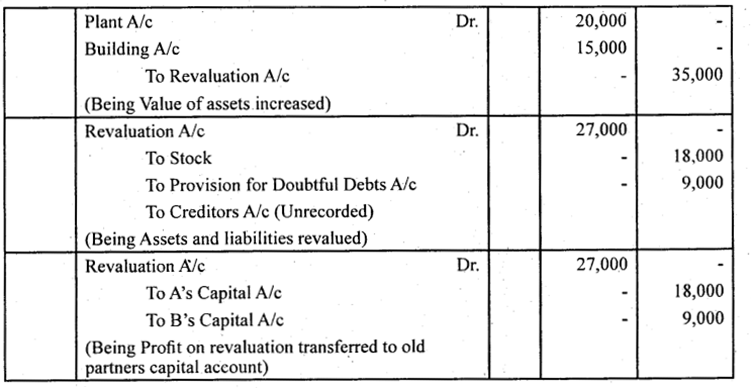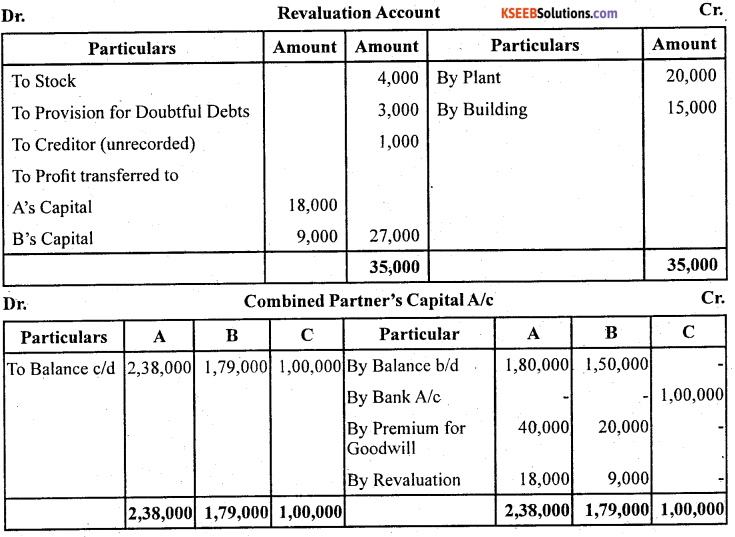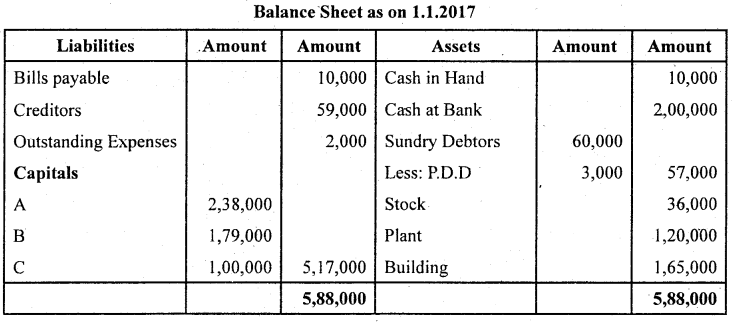Question 28.
LeeLa and Meeta were partners in a firm sharing profits and losses in the ratio 5:3. On 1st Jan. 2007 they admitted Om as a new partner. On the date of Om’s admission the balance sheet of Leela and Meeta showed a balance of ₹ 16,000 in general reserve and ₹ 24,000 (Cr) in Profit and Loss Account. Record necessary journal entries for the treatment of these items on Om’s admission. The new profit sharing ratio between Leela, Meeta and Om was 5:3:2.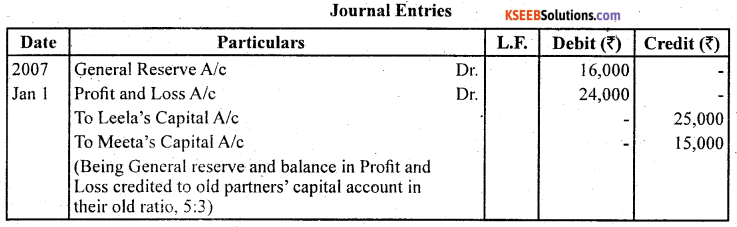Question 29.
Amit and Viney are partners in a firm sharing profits and losses On 1.1.2007 they admitted Ranjan as a partner. On Ranjan’s admission the profit and loss account of Amit and Viney showed a debit balance of ₹ 40,000. Record necessary journal entry for the treatment of the same.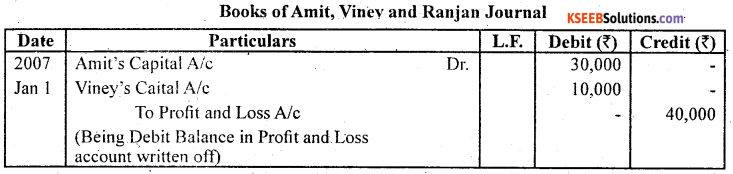Question 29.
A and B share profits in the proportions of 3/4 and 1/4. Their Balance Sheet on 31,2006 was as follows: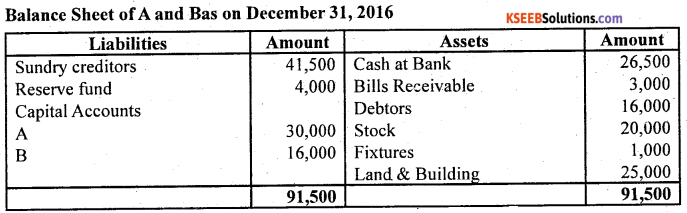On Jan. 1,2017, C was admitted into partnership on the following terms:
(a) That C pays ₹ 10,000 as his capital.
(b) That C pays ₹ 5,000 for goodwill. Half of this sum is to be withdrawn by A and B.
(c) That stock and Fixtures be reduced by 10% and a 5%, provision for doubtful
debts be created on Sundry Debtors and Bills Receivable.
(d) That the value of land and buildings be appreciated by 20%.
(e) There being a claim against the firm for damages, a liability to the extent of Rs 1,000 should be created.
(f) An item of ₹ 650 included in sundry creditors is not likely to be claimed and hence should be written back.
Record the above transactions (journal entries) in the books of the firm assuming that the profit sharing ratio between A and B has not changed. Prepare the new Balance Sheet on the admission of C.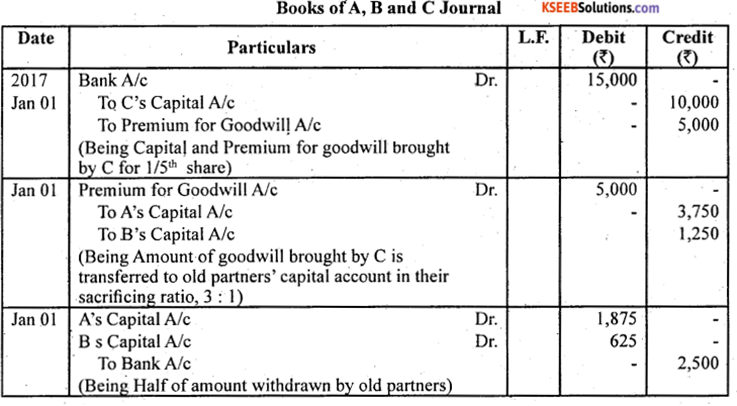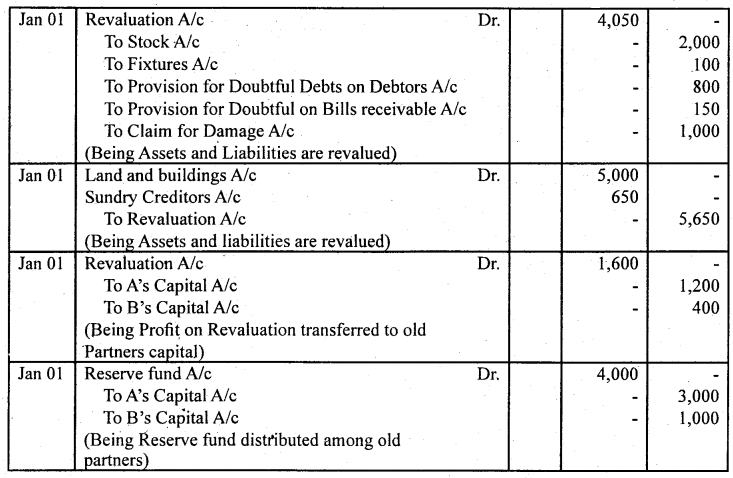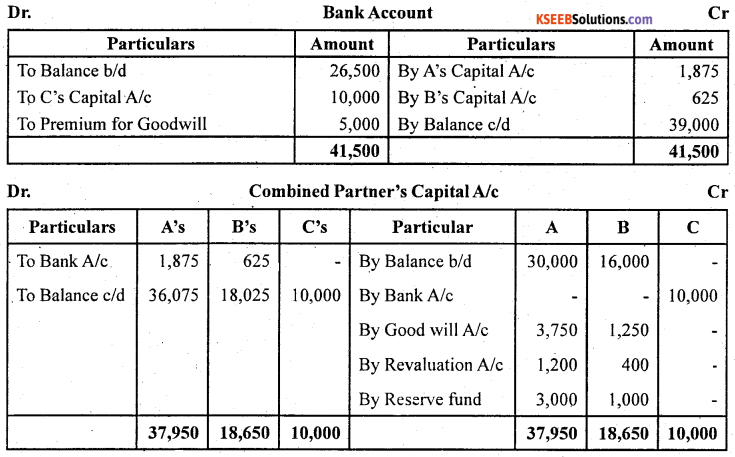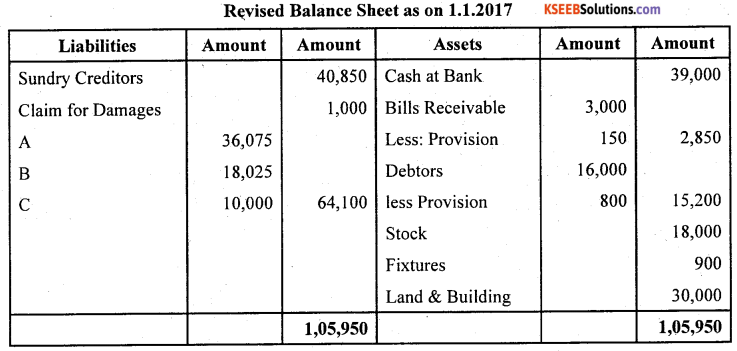Question 30.
A and B are partners sharing profits and losses in the ratio of 3 : 1. On 1st Jan. 2007 the admitted C as a new partner for 1/4 share in the profits of the firm. C brings ₹ 20,000 as for his 1/4 share in the profits of the firm. The capitals of A and B after all adjustments in respect of goodwill, revaluation of assets and liabilities, etc. has been worked out at ₹ 50,000 for A and ₹ 12,000 for B. Its agreed that partner’s capitals will be according to the profit sharing ratio. Calculate the new capitals of A and B and pass the necessary journal entries assuming that A and B brought in or withdrew the necessary cash as the case may be for making their capitals in proportion to their profit sharing ratio?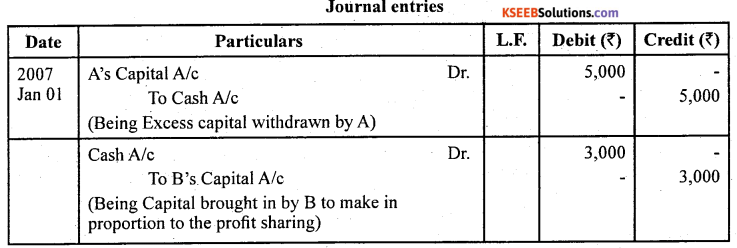1. Calculation of New Profit sharing Ratio
C’s Shares = $$\frac { 1 }{ 4 }$$
Remaining share = $$1-\frac{1}{4}=\frac{3}{4}$$ A’s New share =$$\frac{3}{4} \times \frac{3}{4}=\frac{9}{16}$$
B’s New Share =.$$\frac{1}{4} \times \frac{3}{4}=\frac{3}{16}$$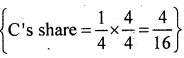New Profit sharing ratio of A; B and C will be 9 : 3 : 4

2. New Capital of A and B.
C bring ₹ 20,000 for 1/4th share of profit in the new firm.
Thus, total capital of firm on the basis of C’s share = 20,000 × $$\frac { 4 }{ 1 }$$ = 80,000
A’s Capital = $$\frac { 9 }{ 16 }$$ × 80,000 = 45,000
Thus, A will withdraw = 50,000 – 45,000 = 5,000
A’s Capital = $$\frac { 3 }{ 16 }$$ × 80,000 = 15,000
Thus, B’s will bring 15,000 – 12,000 = 3,000Question 31.
Roopa partners in a firm sharing profits and losses in the ratio of 3:2:1. S is admitted as a new partner for, 1/4 share in the profits of the firm, which he gets 1/8 from Pinky, and 1/16 each from Qmar and Roopa. The total capital of the new firm after Seema’s admission will be ₹ 2,40,000. Seema is required to bring in cash equal to 1/4 of the total capital of the new firm. The capitals of the old partners also have to be adjusted in proportion of their profit sharing ratio. The capitals of Pinky, Qqmar and Roopa after all adjustments in respect of goodwill and revaluation of assets and liabilities have been made are Pinky ₹ 80,000, Qmar ₹ 30,000 and Roopa ₹ 20,000. Calculate the capitals of all the partners and record the necessary journal entries for doing adjustments in respect of capitals according to the agreement between thee partners?
1. Calculation of new profit sharing Ratio = Old Ratio – Sacrificing Ratio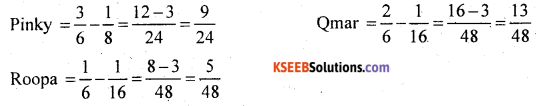New profit sharing ratio between Pinky, Qumar, Roopa and Seema
$$\frac{9}{24}: \frac{13}{48}: \frac{5}{48}: \frac{1}{4}=\frac{18}{48}: \frac{13}{48}: \frac{5}{48}: \frac{12}{48}=18: 13: 5: 12$$

2. Required capital of all partners in the newfirm
18
Pinky’s Capital = 2,40,000 × $$\frac { 18 }{ 48 }$$ = 90,000
Qmar‘s Capital = 2,40,000 × $$\frac { 13 }{ 48 }$$ = 65,000
Roopa’s Capital = 2,40,000 × $$\frac { 5 }{ 48 }$$= 25,000
Seema’s Capital = 2,40,000 × $$\frac { 12 }{ 48 }$$ = 60,000

3. Amount to be brought by each partner
Pinky = 90,000 – 80,000 – 10,000
Qumar= 65,000 – 30,000 = 35,000
Roopa = 25,000 – 20,000 = 5,000
Seema = 2,40,000 × $$\frac { 1 }{ 4 }$$ = 60,000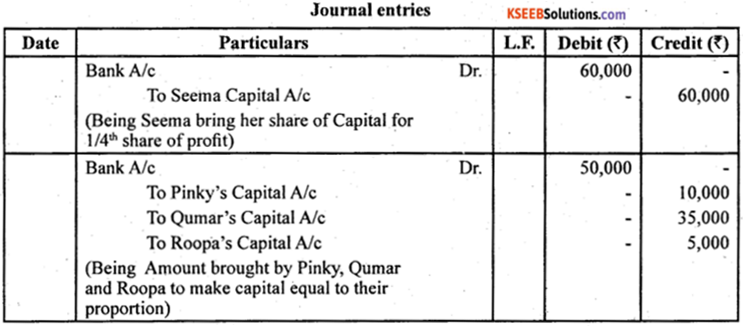Question 32.
The following was the Balance Sheet of Arun, Bablu and Chetan sharing profits and losses in the ratio of $$\frac{6}{14}: \frac{5}{14}: \frac{3}{15}$$ respectively: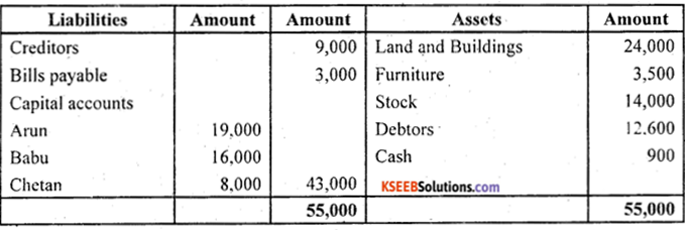They agreed to take Deepak into partnership and give him a share of 1/8 on the following terms:
(a) that Deepak should bring in ₹ 4,200 as goodwill and ₹ 7,000 as his Capital;
(b) that furniture be depreciated by 12%;
(c) that stock be depreciated by 10%;
(d) that a Reserve of 5% be created for doubtful debts;
(e) that the value of land and buildings having appreciated be brought upto? 31,000 (1) that after making the adjustments the capita! accounts of the old partners (who continue to share in the same proportion as before) be adjusted on the basis of the proportion of Deepak’s Capital to his share in the business, i.e., actual cash to be paid off to, or brought in by the old partners as the case may be.

Books of Arun, Bablu, Chetan and Deepak
Prepare Cash Account, Profit and Loss Adjustment Account (Revaluation Account) and the Opening Balance Sheet of the new firm.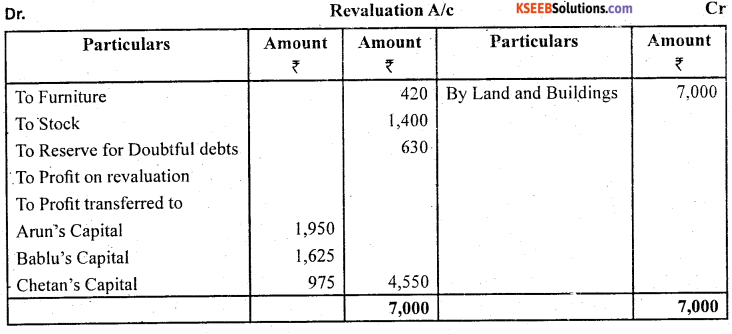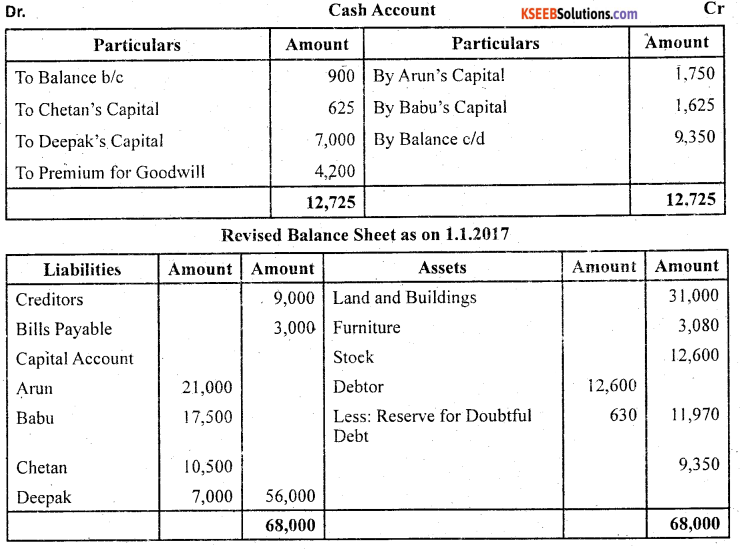Working Note : 1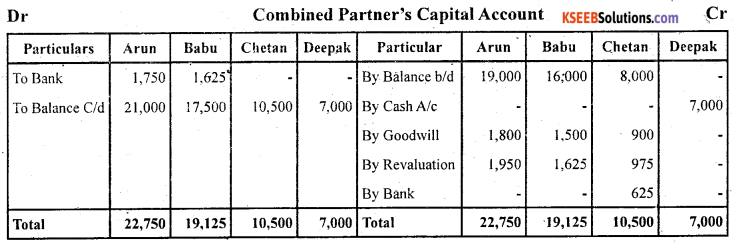Question 33.
Azad and Babli are partners in a firm sharing profits and losses in the ratio of 2:1. Chintan is admitted into the firm with 1/4 share in profits. Chintan will bring in ₹ 30,000 as his capital and the capitals of Azad and Babli are to be adjusted in the profit sharing ratio. The Balance Sheet of Azad and Babli as on December 31, 2006 (before Chinan’admission) was as follows: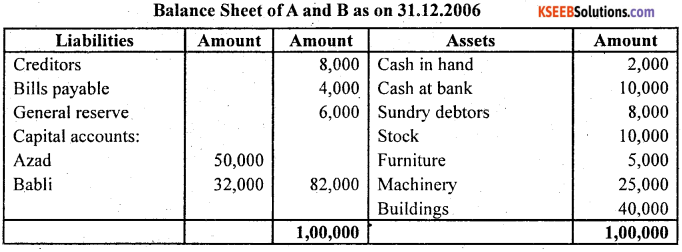It was agreed that;

1. Chintan will bring in ₹ 12,000 as his share of goodwill premium.
2. Buildings were valued at ₹ 45,000 and Machinery at ₹ 23,000.
3. A provision for doubtful debts is to be created’® 6% on debtors.
4. The capital accounts of Azad and Babli are to he adjusted by opening current accounts. Record necessary journal entries, show necessary ledger accounts and prepare the Balance Sheet after admission.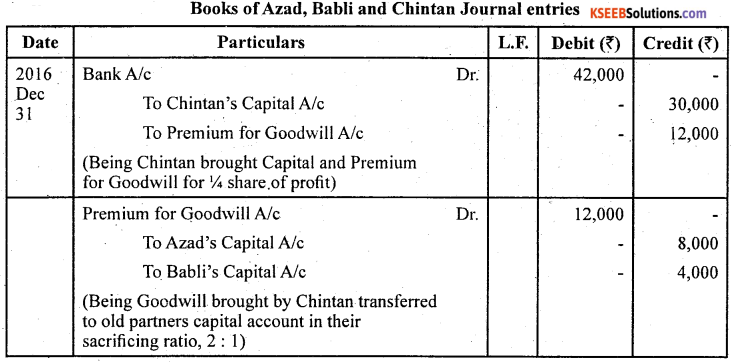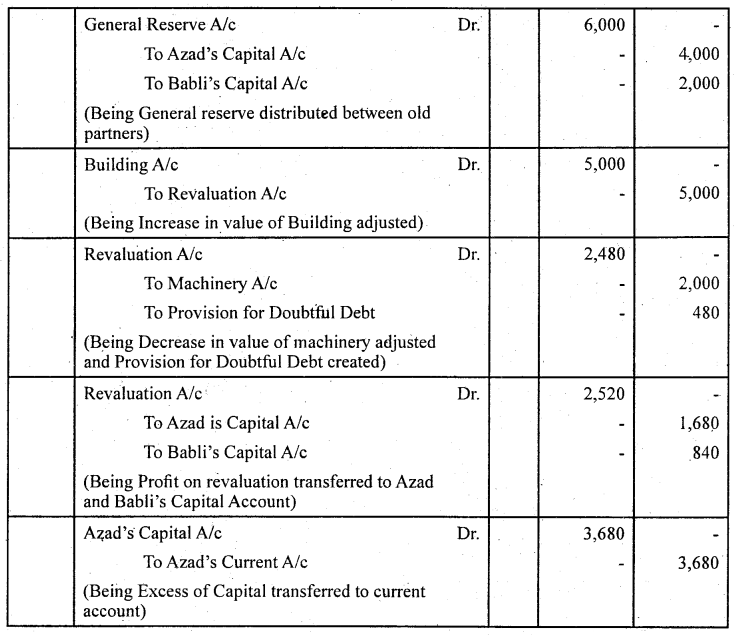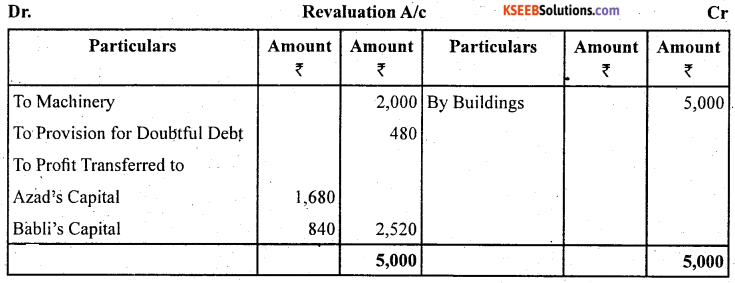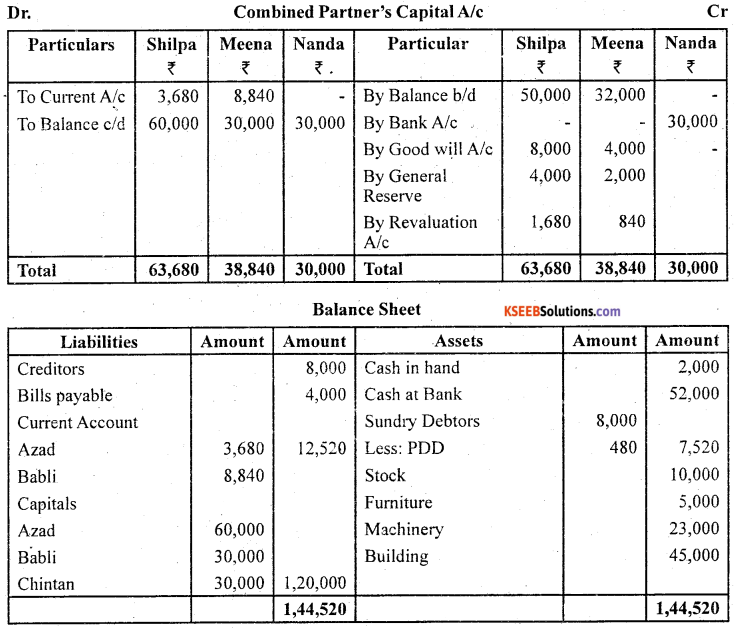Question 34.
Ashish and Dutta were partners in a firm sharing profits in 3:2 ratio. On Jan. 01, 2007 the admitted Vimal for 1/5 share in the profits. The Balance Sheet of Ashish and Dutta as on Jan. 01, 2017 was as follows: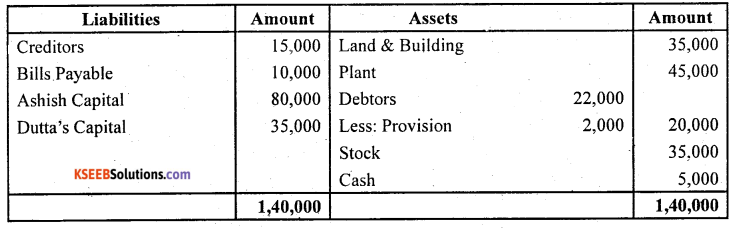It was agreed that:

1. The value of Land and Building be increased by ₹ 15,000.
2. The value of .plant be increased by 10,000.
3. Goodwill of the firm be valued at ₹ 20,000.
4. Vimal to bring in capital to the extent l/5th of the total capital of the new firm. Record the necessary journal entries and prepare the Balance Sheet of the firm after Vimal’s admission.